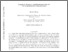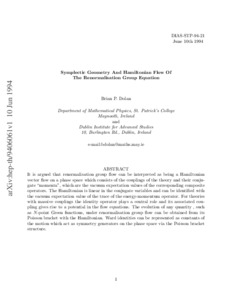# Symplectic geometry and Hamiltonian flow of the renormalisation group equation

Dolan, Brian P. (1995) Symplectic geometry and Hamiltonian flow of the renormalisation group equation. Working Paper. arXiv.Previewmore...Add this article to your Mendeley library

## Abstract

It is argued that renormalisation group flow can be interpreted as being a Hamiltonian vector flow on a phase space which consists of the couplings of the theory and their conjugate \lq\lq momenta", which are the vacuum expectation values of the corresponding composite operators. The Hamiltonian is linear in the conjugate variables and can be identified with the vacuum expectation value of the trace of the energy-momentum operator. For theories with massive couplings the identity operator plays a central role and its associated coupling gives rise to a potential in the flow equations. The evolution of any quantity , such as N-point Green functions, under renormalisation group flow can be obtained from its Poisson bracket with the Hamiltonian. Ward identities can be represented as constants of the motion which act as symmetry generators on the phase space via the Poisson bracket structure.

Item Type: Monograph (Working Paper) Symplectic geometry; Hamiltonian flow; renormalisation group equation; Faculty of Science and Engineering > Mathematical Physics 10506 https://doi.org/10.1142/S0217751X95001273 Dr. Brian Dolan 19 Feb 2019 15:14 arXivItem control page